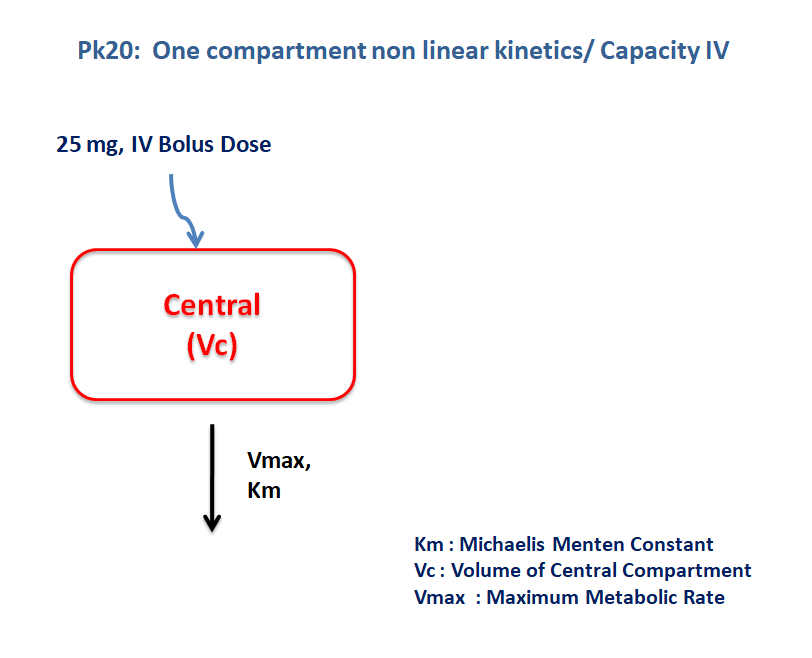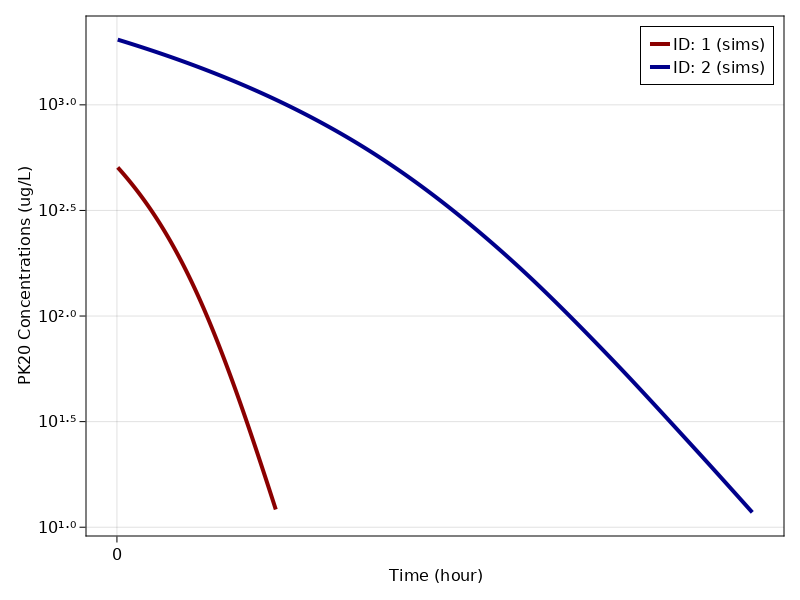# Exercise PK20 - Capacity IV - Nonlinear Kinetics

### Background

• Structural Model - One Compartment Model with Nonlinear elimination.

• Route of administration - IV bolus

• Dosage Regimen - 25 mg, 100 mg

• Number of Subjects - 2### Learing Outcome

This is a one compartment model for IV bolus dose with capacity limited elimination. Concentration time profile was obtained for two subjects with different dosage regimen and different $Vmax$ and $Km$ values.

### Objectives

In this tutorial, you will learn how to build one compartment model for IV bolus dose with capacity limited elimination.

### Libraries

Call the "necessary" libraries to get start.

using Random
using Pumas
using PumasUtilities
using CairoMakie


### Model

pk_20        = @model begin
desc = "One Compartment Model - Nonlinear Elimination"
timeu = u"hr"
end

@param   begin
"Volume of Central Compartment (L)"
tvvc     ∈ RealDomain(lower=0)
"Michaelis menten Constant (μg/L)"
tvkm     ∈ RealDomain(lower=0)
"Maximum Rate of Metabolism (μg/hr)"
tvvmax   ∈ RealDomain(lower=0)
Ω        ∈ PDiagDomain(3)
"Proportional RUV"
σ_prop  ∈ RealDomain(lower=0)
end

@random begin
η        ~ MvNormal(Ω)
end

@pre begin
Vc       = tvvc * exp(η)
Km       = tvkm * exp(η)
Vmax     = tvvmax * exp(η)
end

@dynamics begin
Central' = - (Vmax * (Central/Vc)/(Km + (Central/Vc)))
end

@derived begin
cp       = @. Central/Vc
"""
Observed Concentration (ug/L)
"""
dv       ~ @. Normal(cp, cp*σ_prop)
end
end

PumasModel
Parameters: tvvc, tvkm, tvvmax, Ω, σ_prop
Random effects: η
Covariates:
Dynamical variables: Central
Derived: cp, dv
Observed: cp, dv


### Parameters

The parameters are as given below. tv represents the typical value for parameters.

• $Km$ - Michaelis menten Constant (μg/L)

• $Vc$ - Volume of Central Compartment (L),

• $Vmax$ - Maximum rate of metabolism (μg/hr),

• $Ω$ - Between Subject Variability,

• $σ$ - Residual error

Note:-

• param1 are the parameter values for Subject 1

• param2 are the parameter values for Subject 2

param1 = (tvkm    = 261.736,
tvvmax  = 36175.1,
tvvc    = 48.9892,
Ω       = Diagonal([0.0,0.0,0.0]),
σ_prop = 0.1)

(tvkm = 261.736, tvvmax = 36175.1, tvvc = 48.9892, Ω = [0.0 0.0 0.0; 0.0 0.
0 0.0; 0.0 0.0 0.0], σ_prop = 0.1)

param2 = (param1..., tvkm    = 751.33)

(tvkm = 751.33, tvvmax = 36175.1, tvvc = 48.9892, Ω = [0.0 0.0 0.0; 0.0 0.0
0.0; 0.0 0.0 0.0], σ_prop = 0.1)


We will merge both the parameters

param = vcat(param1, param2)

2-element Vector{NamedTuple{(:tvkm, :tvvmax, :tvvc, :Ω, :σ_prop), Tuple{Flo
at64, Float64, Float64, Diagonal{Float64, Vector{Float64}}, Float64}}}:
(tvkm = 261.736, tvvmax = 36175.1, tvvc = 48.9892, Ω = [0.0 0.0 0.0; 0.0 0
.0 0.0; 0.0 0.0 0.0], σ_prop = 0.1)
(tvkm = 751.33, tvvmax = 36175.1, tvvc = 48.9892, Ω = [0.0 0.0 0.0; 0.0 0.
0 0.0; 0.0 0.0 0.0], σ_prop = 0.1)


### Dosage Regimen

• Subject1 - receives an IV dose of 25 mg or 25000 μg

• Subject2 - receives an IV dose of 100 mg or 100000 μg

ev1  = DosageRegimen(25000, time = 0, cmt = 1)
sub1 = Subject(id = 1, events = ev1, time = 0.01:0.01:2, observations = (cp = nothing,))
ev2  = DosageRegimen(100000, time = 0, cmt = 1)
sub2 = Subject(id = 2, events = ev2, time = 0.01:0.01:8, observations = (cp = nothing,))
pop  = [sub1, sub2]


### Simulation

Simulate the plasma drug concentration for both the subjects

Random.seed!(123)
sim    = map(((subject, paramᵢ),) -> simobs(pk_20, subject, paramᵢ), zip(pop, param))


## Visualization

f, a, p = sim_plot(pk_20, sim,
observations = :cp,
color = :redsblues,
linewidth = 4,
axis = (xlabel = "Time (hour)",
ylabel = "PK20 Concentrations (ug/L)",
xticks = 0:20:160, yscale = log10))
axislegend(a)
f